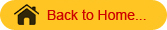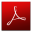## FMP 211 :: Lecture 16 :: COST OF OPERATION OF FARM MACHINERY – PROBLEM SOLVINGUnder cost analysis the cost incurred per hour of operation of a tool/ implement/ machine is calculated. This will give an idea of the pay back period of the investment. This cost serves as the basis to fix up hire charges of the implement for custom hiring
Total cost of operation of an implement/ tool involves two costs namely 1. Fixed cost 2. Variable cost or operating cost
Fixed cost – This cost relates to machine ownership. This cost can occur regardless of whether the machine is used or not. Fixed cost is inversely proportional to the annual use. It includes depreciation, interest on investment, taxes, insurance and housing costs
Variable cost or operating cost – Those costs which are directly related to the amount of use are called variable costs. These costs are incurred only when the machine is used. Variable costs include repair and maintenance, fuel and lubricants, servicing and labour charges.
Calculation of fixed cost

1. Depreciation - It is the reduction in value of the machine with the passage of time. In the usual situation with field machines being operated only a few days in a year year, obsolescence is the most important factor affecting the depreciation (Obsolescence is the state of being which occurs when a person, object, or service is no longer wanted even though it may still be in good working order). A machine may become obsolete because of the development of improved models, changes in farm practices etc.
2. The following expression based on the straight line method is used to calculate the depreciation

D         =          (C   -  S   ﴿    / L ﴿      x   H
Where
D         =          Depreciation cost,   Rs/hr
C         =          Initial cost of the machine,  Rs
S          =          Salvage value of the machine, usually taken as 10
per cent of the initial investment of the machine Rs,
L          =          Expected life period of the machine, years
H         =          Number of working hours per year
Note: Salvage value is the estimated value of an asset at the end of its useful life.
ii. Interest on investment- Interest on investment in a farm machine is a legitimate cost, since money spent in buying a machine cannot be used for other productive enterprises. Annual charges of interest should be calculated on the basis of the actual rate of interest payable. The rate of interest should reflect the prevailing rates (14%).   The interest on investment is calculated by using formula given below.              I           =          (A x   i) / (100 x H) Where,
I           =          Interest on investment, Rs/hr
A          =          Average purchase price, Rs.
H         =          Number of working hours per year
i           =          Rate of interest ( usually it is assumed as 14 % )
The average purchase price shall be calculated by the following expression.                    A          =          (C + S)/2
Where,
A       =        Average purchase price, Rs
C         =          Initial cost of the machine, Rs.
S          =          Salvage value of the machine, usually taken as 10 percent of the
initial investment of the machine,  Rs.
iii.        Taxes, Insurance and housing -. Sales tax and road tax can be distributed over the life of the machine. Farm machinery is sometimes insured against loss by theft or damage.  Actual amount paid or to be paid annually for insurance and annual taxes if any should be charged. If the information is not available it may be calculated on the basis of 2 per cent of the average purchase price per annum. The charge for housing is taken as 1 per cent of the average purchase price of the machine.
So the charges for taxes, insurance and housing can be taken as 3 % per year of the average cost of the machine.

T, I and H        =          3 x A/(100 x H)
Where,
T, I and H        =          Taxes, insurance and housing charges, Rs/hr.
A          =          Average purchase price, Rs.
H         =          Number of working hours per year
The total fixed cost is the sum of depreciation (D), interest on investment (I) and Taxes, Insurance and housing (T, I and H) charges.

2.        Variable cost
i.         Repair and maintenance cost - Repair and maintenance costs are necessary to keep a machine in perfect working condition due to wear, part failure, renewal of tyre and tube and accidents. The repair and maintenance costs shall be calculated as 10 per cent of the initial cost of the machine per year.
R & M  =          10 x C/(100 X H)
Where,
R&M   =          Repair and maintenance costs, Rs/hr
C         =          Initial cost of the machine, Rs.
H         =          Number of working hours per year
ii.         Fuel cost – Fuel consumption depends on the size of the power unit.
The cost of actual fuel consumption    can be used in calculations

Fuel cost  F     = Quantity of fuel consumed per hour (Lit per hour)
(Rs/hr)                         x Cost of fuel (Rs/lit)

iii.        Lubricating oil cost - Cost of lubricating oil can be taken as 30 % of fuel cost

Oil cost = 30 / 100 x Quantity of fuel consumed per hour (Rs/hr)                                            (Lit per hour) x Cost of fuel (Rs/lit)
iv.        Operator cost - In performing custom work, the actual number of operators engaged for carrying out the operation should be used for calculation of operator charges. The prevailing rate of wages has to be adopted for calculation.
Operator cost (Rs/hr) = (Number of persons engaged x wages per  Day)/ 8

The total variable cost is the sum of repair and maintenance cost (R &M), fuel cost, oil cost and operator charges.
Total cost of operation of the = Total fixed cost + Total machine per unit time,
Total cost of operation of the =Total cost of operation of the machine per unit area, Rs/ha machine per unit time, (Rs/hr)/ field capacity of the machine ( ha /hr).
Class work
Problem : The initial cost of 35 hp Massy Ferguson Tractor owned by a farmer is  Rs. 3,00,000/-. The tractor is expected to work for 10 years. In a year the farmer uses the tractor for 1000 hours. The farmer also owns a 11 tined cultivator. The tynes are spaced at 20 cm apart. The cost of the cultivator is Rs.12,000/-. The tractor consumes 3 liters of diesel while ploughing with the cultivator. The life of the cultivator is 10 years. The farmer uses the cultivator for 400 hours in a year.  The cultivator is operated at a speed of 4 km/h. Calculate the cost of ploughing 2 ha of land with the cultivator. Assume all other necessary data.
Calculation
Cost of operation for tractor
1. Depreciation
D         =          (C   -  S   ﴿     / L ﴿       x   H      Where
D         =          Depreciation cost, Rs/hr
C         =          Rs 3,00,000
S          =          10 % of C
L          =          10 years
H         =          1000 hours per year
D         = (3,00,000   -  10/100 (3,00,000 ﴿     / 10 x     1000 ﴿
= Rs. 27 / hour

2. Interest I
Average cost A
A          =          (3,00,000 + 30,000)/2 =       Rs. 1,65,000
I           =          (1,65,000 x   14) / (100 x 1000)
Rs. 23.1

3. Taxes, Insurance and housing
T, In and H            =          3 x 1,65,000/(100 x 1000)
=       Rs. 4.95
Total fixed cost = 27 + 23.1 + 4.95
=  Rs. 55.05 / h
Variable cost
1. Repair and maintenance
R & M                    =          10 x 3,00,000/(100 X 1000)
=        Rs. 30/h
2. Fuel cost F       =          3.0 lit/h x Rs 45/lit
=          Rs. 135/lit
3. Lubricating oil cost    = (3 0/100 ) x Rs. 135/lit
=  Rs. 40.5 / h
4.Operator cost (Rs/hr) = ( 1 person x Rs.240/day )/ 8
= Rs. 30 / h
Total variable cost    = 30 +135 + 40.5 + 30
= Rs. 235 / h
Cost of operation for tractor
Total fixed cost + Total variable cost
Rs. 55.05 + Rs. 235 = Rs. 290.05 / h
Cost of operation for implement
1. Depreciation
D         =          (C   -  S   ﴿     / L ﴿       x   H Where
D         =          Depreciation cost, Rs/hr
C         =          Rs 12,000
S          =          10 % of C
L          =          10 years
H         =          400 hours per year
D         =          (12,000 -  10/100 (12,000 ﴿ / 10 x     400 ﴿
=           Rs. 2 .7 / hour

2. Interest  I
Average cost A
A          =          (12,000 + 1,200)/2
=       Rs. 6,600
I           =          (6,600 x   14) / (100 x 400)
Rs. 2.31/h
3. Taxes, Insurance and housing
T, In and H =         3 x 6,600/(100 x 400)
=       Rs. 0.495 /h
Total fixed cost = 2 .7 + 2.31 + 0.495
=  Rs.5.51 / h
Variable cost
1. Repair and maintenance
R & M                    =          10 x 12,000/(100 X 400)
=        Rs. 3 / h
Lubricating oil cost    =  Nil
Operator cost (Rs/hr) =  Nil
Total variable cost =  Rs. 3 / h
Cost of operation for implement
Total fixed cost + Total variable cost
Rs.5.51 + Rs.3 =  Rs. 8.51
Cost of operation for tractor and implement
Rs. 290.05 / h + Rs. 8.51/h = Rs. 298.55/h
Field capacity of implement
FC =S W/10 ha/h
= (2.2 x 4.0) / 10 = 0.88 ha/h
Time required to complete 1.0 ha land
= 1/ field capacity
= 1/ 0.88
= 1.136 hour/ha
Cost of ploughing 1.0 ha land = No. of hours / ha x
cost of ploughing Rs./ ha
= 1.136 x Rs. 298.55
= Rs. 339.16/ha

Average Field Speeds, Field Efficiencies, and Effective Field Capacities 0f some farm machinery:

 Machine Size Speed (mph) Field Efficiency (%) Effective Field Capacity (A/h) Fertilizer Spreader 40' 6 70 20.4 50' 6 70 25.5 Manure Spreader 10' 5 63 3.8 Anhydrous Ammonia Applicator 9 knife 5 65 8.9 Plow 7–16" 5 85 4.8 Subsoiler 5–24" 5 85 5.2 Chisel Plow 11’3" 5.5 85 6.4 Offset Disk 12' 5.5 85 6.8 Tandem Disk 14' 6 83 8.5 Field Cultivator/Seedbed Conditioner 15' 7 85 10.8 Planter, seed only 6–30" 5 65 5.9 Grain or Soybean Drill 10' 6 70 5.1 Broadcast Seeder 20' 5 70 8.5 Sprayer 20' 6 65 9.5 Rotary Hoe Row-crop Cultivator 6–30" 4 80 5.8 Mower Conditioner rotary 9' 7 83 6.3 cutterbar 12' 5 80 5.8 Combine, soybeans* 15' 3.8 73 5.0 self-propelled Forage Harvester 3 rows 25

Problems
1. Calculate the cost of seeding one hectare of land with bullock drawn seed drill of size 5 x 22 cm. the speed of bullocks is 3 km/h. Hire charges of bullocks is Rs. 150/day.. Hire charges of seed drill is Rs. 100/day of 8 hours.

2. A flutted feed seed drill has 8 furrow openers of single disc type. The furrow openers are spaced 25 cm apart and the main drive wheel has a diameter of 120 cm. How many turns of main drive wheel would occur when the seed drill has covered 1.0 ha of land.Download this lecture as PDF here